# Math vocabulary. Lessons 1-5Page 1

#### WATCH ALL SLIDES

Slide 1Vocabulary Quiz Review

Lessons 1-5

Saxon7/6

Slide 2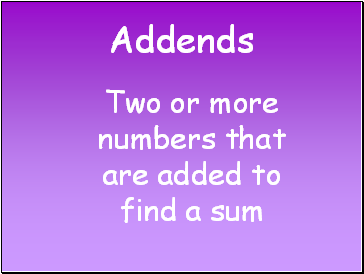Two or more numbers that are added to find a sum

Slide 3Changing the order of addends does not change their sum.

Ex. A + B = B + A

Slide 4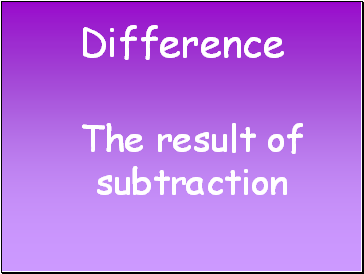## Difference

The result of subtraction

Slide 5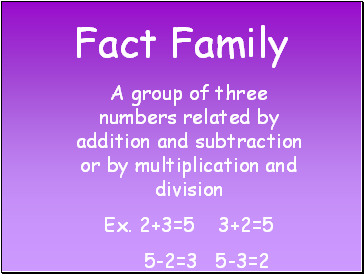## Fact Family

A group of three numbers related by addition and subtraction or by multiplication and division

Ex. 2+3=5 3+2=5

5-2=3 5-3=2

Slide 6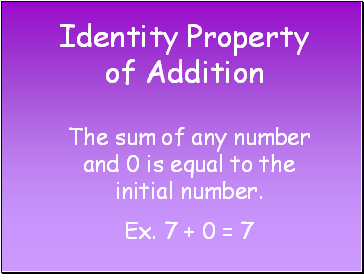The sum of any number and 0 is equal to the initial number.

Ex. 7 + 0 = 7

Slide 7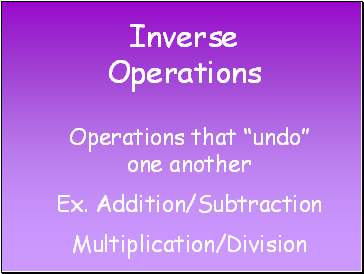## Inverse Operations

Operations that “undo” one another

Multiplication/Division

Slide 8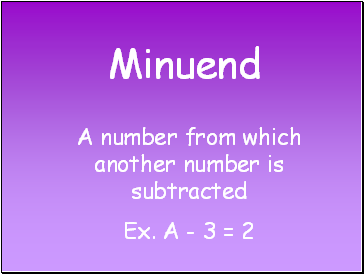## Minuend

A number from which another number is subtracted

Ex. A - 3 = 2

Slide 9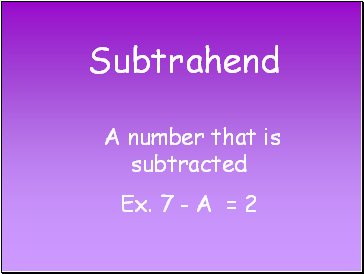## Subtrahend

A number that is subtracted

Ex. 7 - A = 2

Slide 10## Sum

Slide 11## Commutative Property of Multiplication

Changing the order of factors does not change their product.

Ex. A x B = B x A

Slide 12## Dividend

A number that is divided

Slide 13## Divisor

A number by which another number is divided

Slide 14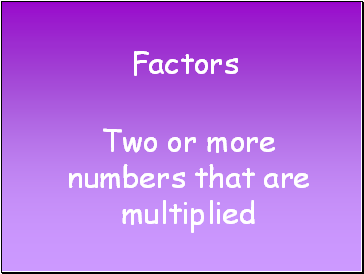## Factors

Two or more numbers that are multiplied

Slide 15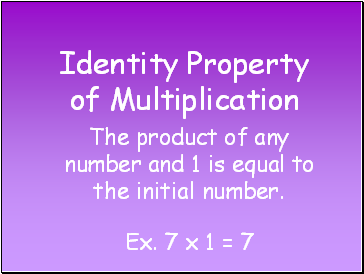## Identity Property of Multiplication

The product of any number and 1 is equal to the initial number.

Ex. 7 x 1 = 7

Slide 16## Product

The result of multiplication

Go to page:
1  2